Courses

# 28 Year NEET Questions: Some Basic Principles And Techniques- 3

## 30 Questions MCQ Test Chemistry 31 Years NEET Chapterwise Solved Papers | 28 Year NEET Questions: Some Basic Principles And Techniques- 3

Description
This mock test of 28 Year NEET Questions: Some Basic Principles And Techniques- 3 for NEET helps you for every NEET entrance exam. This contains 30 Multiple Choice Questions for NEET 28 Year NEET Questions: Some Basic Principles And Techniques- 3 (mcq) to study with solutions a complete question bank. The solved questions answers in this 28 Year NEET Questions: Some Basic Principles And Techniques- 3 quiz give you a good mix of easy questions and tough questions. NEET students definitely take this 28 Year NEET Questions: Some Basic Principles And Techniques- 3 exercise for a better result in the exam. You can find other 28 Year NEET Questions: Some Basic Principles And Techniques- 3 extra questions, long questions & short questions for NEET on EduRev as well by searching above.
QUESTION: 1

### The number of possible isomers of the compound with molecular formula C7 H8O is 

Solution:

The possible isomers of the compound with molecular formula C7H8O is 5. These are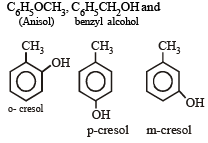QUESTION: 2

### The IUPAC name of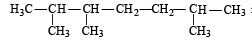Solution:

When many substituents are present, the numbering is done from the end where the sum of locants is the lowest (lowest sum rule)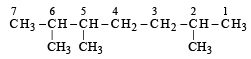2, 5, 6 trimethyl heptane (wrong) 2 + 5 + 6 = 13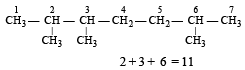2, 3, 6 TriMethyl Heptane (correct)

QUESTION: 3

### Huckel's rule states that a monocyclic conjugated compound will be aromatic if it contains 

Solution:

Huckel’s rule states that for aromaticity there must be (4n + 2)π electron present in compound where n is an integer

QUESTION: 4

Which of the following will not show cis-trans isomerism? 

Solution: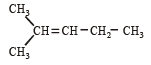Due to presence two similar methyl group at same carbon atom, above compound doesn’t show geometrical isomerism.

QUESTION: 5

Which of the following will exhibit chirality? 

Solution: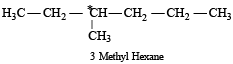Due to presence of four different groups on carbon,(C*) it is chiral

QUESTION: 6

Decreasing order of reactivity to wards nucleophilic addition to carbonyl group among cyclopentanone, 3-pentanone and n-pentanal is 

Solution:

More is the electron - dificiency of the carbonyl carbon, greater will be the reactivity of the carbonyl compounds towards nucleophilic addition.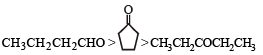QUESTION: 7

Which one of the following is a technique most suitable for purification of cyclohexanone from a mixture containing benzoic acid, isoamyl alcohol, cyclohexane and cyclohexanone? 

Solution:

IR spectroscopy is used for the purification of cyclohexanone from a mixture of benzoic acid, isoamyl alcohol, cyclohexane and cyclohexanone. Because in this method, each functional group uppear at a certain peak.
So, cyclohexanone can be identified by carbonyl peak.

QUESTION: 8

Tautomerism will be exhibited by 

Solution:

Tautomerism is exhibited by  the oscillation of hydrogen atom between two polyvalent atoms present in the molecule. As option (d) has α-hydrogen atom.  Therefore it shows tautomerism whereas other structures do not.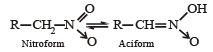QUESTION: 9

The reaction :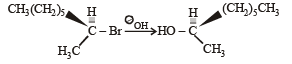is described as 

Solution:

When (–) 2 bromo octane is allowed to react with sodium hydroxide under given conditions, where second order kinetics are followed, the product obtained is (+) 2 octanol.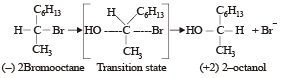In this reaction Walden Inversion takes place so it is an example of SN 2-reaction.

QUESTION: 10

Which one of these is not compatible with arenes? 

Solution:

In arenes, π electrons are delocalised, hence arenes do not undergo addition reactions easily. Aromatic compounds (Arenes) are highly stable and show resorance. eg. Benzene is the simplest example.

QUESTION: 11

The most stable conformation of n-butane is

Solution:

Order of stability : staggered anti > gauche > skew boat > eclipsed.
Newmann projection of n butane is given as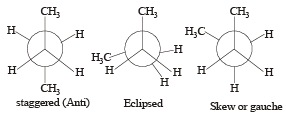The maximum staggered conformation is most stable in which methyl groups are far apart as for as possible, due to minimum repulsion between methyl groups and is also called anti conformation.

QUESTION: 12

Among the following compounds (I - III), the ease of their reaction with electrophiles is,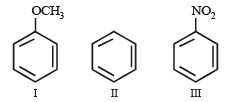Solution:

–OCH3 activates the benzene ring. –NO2 deactivates the ring. Hence the reaction of the given compounds with electrophiles is in the order, I > II > III

QUESTION: 13

IUPAC name for the compound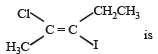Solution: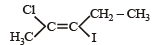As in this compound the common groups i.e highly electronegative halogen atoms are on opposite side, hence it is a trans isomer.
Thus its name is trans-2-chloro-3-iodo-2-pentene.

QUESTION: 14

Which one of the following compounds will be most easily attacked by an electrophile? 

Solution:

—Cl atom sh ows o/p-dir ective influence but deactivate the benzene ring, while [—OH/—CH3] groups show o/p  influence but activate the benzene ring but in these —OH group is more activating than —CH3.
Hence order of electrophilic substitution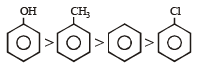QUESTION: 15

Which of the following compounds is not chiral? 

Solution:

Due to absence of a symmetric (chiral) C-atom. D — CH2 — CH2 — CH2Cl molecule is not a chiral molecule.

QUESTION: 16

Which one of the following compounds is resistant to nucleophilic attack by hydroxyl ions?

Solution:

The compound is diethyl ether (CH3CH2)2O which is resistant to nucleophilic attack by hydroxyl ion due to absent of double or triple bond, whereas all other compounds given are unsaturated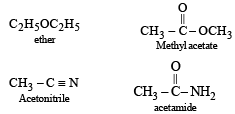QUESTION: 17

Which one of the following orders is correct regarding the —I effect of the substituents ?

Solution:

The atom or group which has more power to attract electrons in comparision to hydrogen is said to have -I effect. Thus higher the electronegativity of atom stronger will be the -I effect. As electronegativity of N, O & F follow the order N < O < F hence  based upon electronegative character order of-I effect is – NR2 < – OR < – F.

QUESTION: 18

The correct structure of trans-2 hexenal is  

Solution:

When similar atoms are on opposite side the compound is in trans-form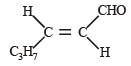QUESTION: 19

The structural formula of a compoun d is CH3 – CH = C = CH2. The types of hybridization at the four carbons from left to right are

Solution: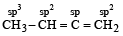QUESTION: 20

Which is a chiral molecule? 

Solution:

Chiral molecules are those molecules which have atleast one symmetric carbon atom (a carbon atom attached to 4 different groups).
This is true in case of 3-methyl pentanoic acid.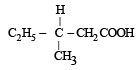QUESTION: 21

Consider the following phenols :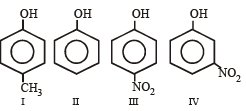The decreasing order of acidity of the above phenols is 

Solution:

Electron with drawing group (–NO2) increases acidity while electron releasing group (–CH3, –H) decreases acidity. Also effect will be more if functional group present at para position then ortho and meta position.

QUESTION: 22

Correct order of stability is : 

Solution:

Stability of an alkene depends upon the heat of hydrogenation of an alkene. The lower the heat of hydrogenation of an alkene higher will be stability.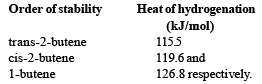QUESTION: 23

Which of the following compounds reacts slower in electrophilic substitution? 

Solution:

CH3 and NH2 are electron repelling groups, whereas NO2 and OH are electron attracting groups and they leave the benzene ring deactivated. Due to stronger electron attracting (–I effect) effect of NO2 group C6H5NO2 shows least reactivity towards electrophilic substitution.

QUESTION: 24

A compound of molecular formula of C7H16 shows optical isomerism, compound will be

Solution:

A compound is said to exhibi top tical isomerism if it atleast contains one chiral carbon atom, which is an atom bonded to 4 different atoms or groups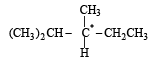QUESTION: 25

The correct order of acidic strength for following compounds will be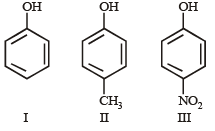Solution:

Electron releasing group (–CH3)decreases acidity while electron withdrawing group (– NO2)increases acidity.

QUESTION: 26

In steam distillation of toluene, the pressure for toluene in vapour is 

Solution:

The principle of steam distillation is based on Dalton's law of partial pressures. Suppose p1 and p2 be the vapour pressures of water vapour and the toluene at the distillation temperature. Toluene boils  when total pressure is equal to atmospheric pressure p i.e p  = p1 + p2 or  p2 = p – p1 as a result when toluene boil in  the presence of steam. Its partial pressure p2 is less than atmospheric pressure.

QUESTION: 27

The incorrect IUPAC name is 

Solution: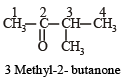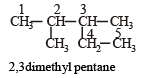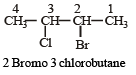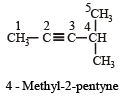QUESTION: 28

IUPAC name of the following is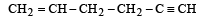Solution:

The IUPAC name of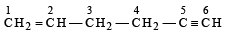is Hex-1-en-5-yne or 1-hexene-5-yne The lowest number is given to the C = C double bond.

QUESTION: 29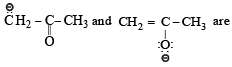Solution: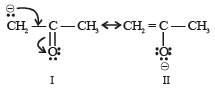both are resonating structures.

QUESTION: 30

Geometrical isomers differ in 

Solution:

Geometrical isomers differ in spatial arrangement of atoms.